Courses

# Lakhmir Singh & Manjit Kaur: Magnetic Effects of Electric Current, Solutions- 2 Class 10 Notes | EduRev

## Class 10 : Lakhmir Singh & Manjit Kaur: Magnetic Effects of Electric Current, Solutions- 2 Class 10 Notes | EduRev

The document Lakhmir Singh & Manjit Kaur: Magnetic Effects of Electric Current, Solutions- 2 Class 10 Notes | EduRev is a part of the Class 10 Course Lakhmir Singh & Manjit Kaur Solutions: Class 10 Science.
All you need of Class 10 at this link: Class 10

Page No:81

Question 1: Which effect of current can be utilised in detecting a current­carrying wire concealed in a wall ?

Solution : Magnetic effect.

Question 2: What conclusion do you get from the observation that a current-carrying wire deflects a compass needle placed near it ?

Solution : We conclude that a current carrying wire produces a magnetic field around it.

Lakhmir Singh Biology Class 10 Solutions Page No:82

Question 3: Name the scientist who discovered the magnetic effect of current.

Solution : Magnetic effect of current was discovered by Oersted.

Question 4: State qualitatively the effect of inserting an iron core into a current-carrying solenoid.

Solution : Magnetic field becomes very strong.

Question 5: Name the rule for finding the direction of magnetic field produced by a straight current-carrying conducloj.

Solution : Maxwell’s right hand thumb rule.

Question 6: State the form, of magnetic field lines around a straight current-carrying conductor.

Solution : The magnetic field lines around a straight current-carrying conductor are concentric circles whose centres lie on the conductor.

Question 7: What is the other name of Maxwell’s right-hand thumb rule ?

Solution : Maxwell’s corkscrew rule.

Question 8: State whether the following statement is true or false :
The magnetic field inside a long circular coil carrying current will be parallel straight lines.

Solution : True.

Question 9: What is the shape of a current-carrying conductor whose magnetic field pattern resembles that of a bar magnet ?

Solution : Solenoid.

Question 10: State three ways in which the strength of an electromagnet can be increased.

Solution :

The strength of an electromagnet can be increased by
(i) increasing the number of turns in the coil
(ii) increasing the current flowing in the coil
(iii) reducing the length of air gap between the poles

Question 11:
Fill in the following blanks with suitable words :
(a) The lines of……………. round a straight current-carrying conductor are in the shape of…………………………
(b) For a current-carrying solenoid, the magnetic field is like that of a………………………….
(c) The magnetic effect of a coil can be increased by increasing the number of………………………. , increasing the…………….. , or inserting an…………….. core.
(d) If a coil is viewed from one end and the current flows in an anticlockwise direction, then this end is a pole.
(e) If a coil is viewed from one end, and the current flows in a clockwise direction, then this end is a…………………..

Solution :
(a) magnetic field; concentric circles
(b) bar magnet
(c) turns; current; iron
(d) north
(e) south.

Question 12: Describe how you will locate a current-carrying wire concealed in a wall.

Solution : A current-carrying wire concealed in a wall can be located due to the magnetic effect of current by using a plotting compass. If a plotting compass is moved on a wall, its needle will show deflection at the place where current-carrying wire is concealed.

Question 13: Describe some experiment to show that the magnetic field is associated with an electric current.

Solution :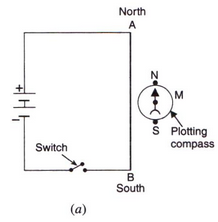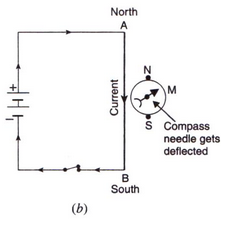We take a thick insulated copper wire and fix it in such a way that the portion AB of the wire is in the north-south direction as shown in fig. A plotting compass M is placed under the wire AB. The two ends of the wire are connected to a battery through a switch. When no current is flowing in the wire AB, the compass needle is parallel to the wire AB and points in the usual north-south direction. When current is passed through wire AB by closing the switch, we find that the compass needle is deflected from its north-south position. On opening the switch, the compass needle returns to its original position.
Thus, the deflection of compass needle by the current carrying wire shows that magnetic field is associated with an electric current.

Question 14: (a) Draw a sketch to show the magnetic lines of force due to a current-carrying straight conductoi
(b) Name and state the rule to determine the direction of magnetic field around a straight current-oar- ing conductor.

Solution :

(a)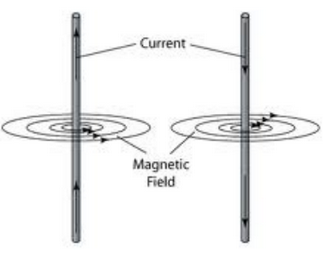(b) Maxwells right-hand thumb rule: According to this rule, imagine that you are grasping the current-carrying wire in your right hand so that your thumb points in the direction of current, then the direction in which your fingers encircle the wire will give the direction of magnetic field lines around the wire.

Question 15: State and explain Maxwell’s right-hand thumb rule.

Solution : According to Maxwell’s right hand thumb rule: Imagine that you are grasping the current-carrying wire in your right hand so that your thumb points in the direction of current, then the direction in which your fingers encircle the wire will give the direction of magnetic field lines around the wire.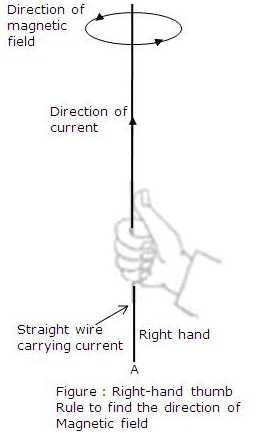Let AB be the straight wire carrying current in the vertically upward direction from A to B. To find out the direction of the magnetic field lines produced by this current, we imagine that we are grasping the current carrying wire in our right hand as shown in fig. so that our thumb points in the direction of current towards B. Now, the direction in which our fingers are folded gives the direction of magnetic field lines. In this case, the direction of magnetic field lines is in the anticlockwise direction.

Question 16: What is Maxwell’s corkscrew rule ? For what purpose is it used ?

Solution : According to Maxwell’s corkscrew rule: Imagine driving a corkscrew in the direction of current, then the direction in which we turn its handle is the direction of magnetic field.
This rule is used to determine the direction of magnetic field around a straight current carrying conductor.

Question 17: (a) Draw the magnetic lines of force due to a circular wire carrying current.
(b) What are the various ways in which the strength of magnetic field produced by a current-carrying circular coil can be increased ?

Solution :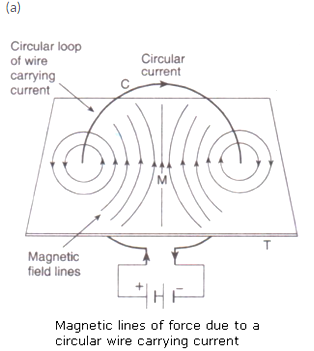(b) The strength of magnetic field produced by a current-carrying circular coil can be increased by:
-increasing the number of turns of wire in the coil.
-increasing the current flowing through the coil.

Question 18: State and explain the Clock face rule for determining the polarities of a circular wire carrying current.

Solution : According to the Clock face rule, we look at one face of a circular wire (or coil) through which a current is passing:
(i) If the current around the face of the circular wire (or coil) flows in the clockwise direction, then that face of the circular wire will be South pole (S-pole).
(ii) If the current around the face of the circular wire (or coil) flows in the anti-clockwise direction, then that face of the circular wire will be North pole (N-pole).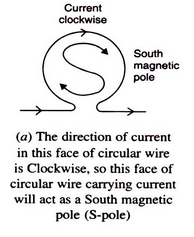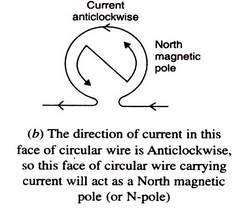Question 19: Name any two factors on which the strength of magnetic field produced by a current-carrying solenoid depends. How does it depend on these factors ?

Solution : The strength of magnetic field produced by a current-carrying solenoid depends on:
1. The strength of current in the solenoid: Larger the current passed through solenoid, stronger will be the magnetic field produced.
2. The number of turns in the solenoid: Larger the number of turns in the solenoid, greater will be the magnetic field produced.

Question 20: (a) Draw a circuit diagram to show how a soft iron piece can be transformed into an electromagnet.
(b) Describe how an electromagnet could be used to separate copper from iron in a scrap yard.

Solution :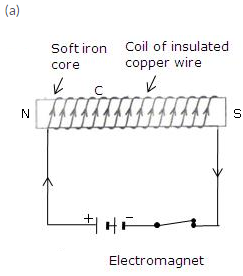A coil C of insulated copper wire is wound around a soft iron core NS and the two ends of the copper coil are connected to a battery. Thus, an electromagnet using a soft iron core.
(b) Electromagnetic cranes are used to separate copper from iron in a scrap yard. The current is switched on to energise the electromagnet and pick up the iron pieces from the scrap. Then these iron pieces are moved to another position, the electromagnet in switched off and the iron pieces are released.

Question 21: (a) How does an electromagnet differ from a permanent magnet ?
(b) Name two devices in which electromagnets are used and two devices where permanent magnets are used.

Solution : (a) An electromagnet produces a magnetic field so long as current flows in its coil i.e., it produces temporary magnetic field.; but a permanent magnet produces a permanent magnetic field.
(b) Electromagnets: Electric bell, electric motors
Permanent magnets: Refrigerator doors, toys

Question 22: (a) What is a solenoid ? Draw a sketch to show the magnetic field pattern produced by a current-carrying
solenoid.
(b) Name the type of magnet with which the magnetic field pattern of a current-carrying solenoid resembles.
(c) What is the shape of field lines inside a current-carrying solenoid ? What does the pattern of field lines inside a current-carrying solenoid indicate ?
(d) List three ways in which the magnetic field strength of a current-carrying solenoid can be increased ?
(e) What type of core should be put inside a current-carrying solenoid to make an electromagnet ?

Solution :
(a) A solenoid is a long coil containing a large number of close turns of insulated copper wire.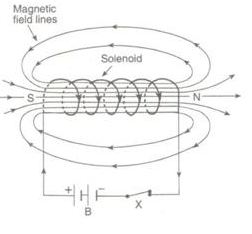(b) The magnetic field produced by a current-carrying solenoid is similar to the magnetic field produced by a bar magnet.
(c) Magnetic field lines inside a current-carrying solenoid are in the form of parallel straight lines. This indicates that the magnetic field inside the solenoid is uniform.
(d) The magnetic field strength of a current-carrying solenoid can be increased by
(i) increasing the number of turns in the solenoid.
(ii) increasing the current flowing through the solenoid.
(iii) using soft iron as core in the solenoid.
(e) Soft iron core.

Question 23: (a) What is an electromagnet ? Describe the construction and working of an electromagnet with the help of
a labelled diagram.
(b) Explain why, an electromagnet is called a temporary magnet.
(c) Explain why, the core of an electromagnet should be of soft iron and not of steel.
(d) State the factors on which the strength of an electromagnet depends. How does it depend on these factors ?
(e) Write some of the important uses of electromagnets.

Solution :
(a) An electromagnet is a temporary magnet that works on the magnetic effect of current. It consists of a long coil of insulated copper wire wrapped around a soft iron core that is magnetised ony when electric current is passed through the coil.
To make an electromagnet, we take a rod NS of soft iron and wind a coil C of insulated copper wire around it. When the two ends of the copper coil are connected to a battery, an electromagnet is formed.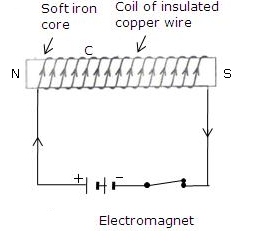(b) An electromagnet is called a temporary magnet because it produces magnetic field so long as current flows in its coil.
(c) Core of an electromagnet should be of soft iron and not of steel because soft iron loses all its magnetism when current in the coil is switched off but steel does not lose its magnetism when the current is stopped.
(d) Strength of electromagnet depends on:
i. The number of turns in the coil – Increasing the number of turns in the coil increases the strength of the electromagnet.
ii. The current flowing in the coil – Increasing the current flowing in the coil increases the strength of the electromagnet.
iii. The length of air gap between its poles: Reducing the length of air gap between the poles of electromagnet increases the strength of the electromagnet.
(e) Electromagnets are used in several electrical devices such as electric bell, electric motor, loudspeaker etc. They are also used by doctors to remove particles of iron or steel from a patient’s eye and to remove pieces of iron from wounds.

Page No:84

Question 37: In the straight wire A, current is flowing in the vertically downward direction whereas in wire B the current is flowing in the vertically upward direction. What is the direction of magnetic field :
(a) in wire A ?
(b) in wire B ?
Name the rule which you have used to get the answer.

Solution :

(a) Clockwise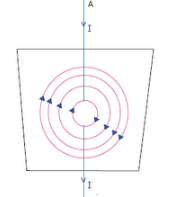(b) Anticlockwise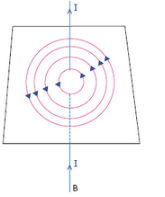We have used Maxwell's right hand thumb rule here

Question 38: The figure shows a solenoid wound on a core of soft iron. Will the end A be a N pole or S pole when the current flows in the direction shown ?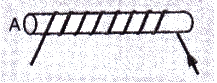Solution : End A will be a S-pole because current flows in the clockwise direction at A.

Question 39: A current-carrying straight wire is held in exactly vertical position. If the current passes through this wire in the vertically upward direction, what is the direction of magnetic field produced by it ? Name the rule used to find out the direction of magnetic field.

Solution : Direction of magnetic field is anticlockwise.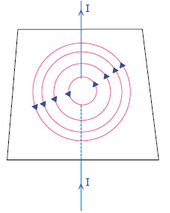Maxwell’s right hand thumb rule. is used to find out the direction of magnetic field.

Question 40: For the coil in the diagram below, when the switch is pressed :
(a) what is the polarity of end A ?
(b) which way will the compass point then ?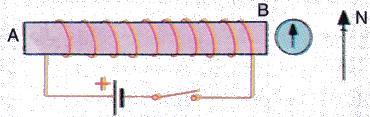Solution : (a) End A becomes S-pole because current flows in clockwise direction at A.
(b) When A becomes S-pole, the other end becomes N-pole. So the tip of the compass (with also has North polarity) moves away from this end i.e., tip moves towards right.

Question 41: A current flows downwards in a wire that passes vertically through a table top. Will the magnetic field lines around it go clockwise or anticlockwise when viewed from above the table ?

Solution : Magnetic field lines around it will be clockwise (according to Maxwell’s right hand thumb rule).

Question 42: The directions of current flowing in the coil of an electromagnet at its two ends X and Y are as shown below:
(a) What is the polarity of end X ?
(b) What is the polarity of end Y ?
(c) Name and state the rule which you have used to determine the polarities.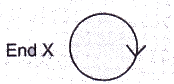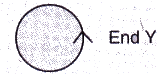Solution : (a) End X is S-pole (because current flows in clockwise direction).
(b) End Y is N-pole (because current flows in anticlockwise direction).
(c) Clock face rule – Looking at the face of a loop, if the current around that face is in anticlockwise direction, the face has north polarity, while if the current at that face is in clockwise direction, the face has south polarity.

Question 43: The magnetic field associated with a current-carrying straight conductor is in anticlockwise direction. If the conductor was held along the east-west direction, what will be the direction of current through it ? Name and state the rule applied to determine the direction of current ?

Solution : The direction of current will be from east to west.
We have applied MAxwell’s right hand thumb rule here.
According to Maxwell’s right hand thumb rule: Imagine that you are grasping the current-carrying wire in your right hand so that your thumb points in the direction of current, then the direction in which your fingers encircle the wire will give the direction of magnetic field lines around the wire.

Question 44: A current-carrying conductor is held in exactly vertical direction. In order to produce a clockwise magnetic field around the conductor, the current should be passed in the conductor :
(a) from top towards bottom
(b) from left towards right
(c) from bottom towards top
(d) from right towards left

Solution : (a) from top towards bottom.

Question 45: A thick wire is hanging from a wooden table. An anticlockwise magnetic field is to be produced around the wire by passing current through this wire by using a battery. Which terminal of the battery should be connected to the :
(a) top end of wire ?
(b) bottom end of wire ?
(c) Give reason for your choice.

Solution : (a) Negative terminal
(b) Positive terminal
Because the current should be passed into wire upwards.

Page No:85

Question 1: What produces magnetism in the human body ?

Solution : Weak ionic currents flowing in the human body produce magnetism.

Question 2: Name one medical technique which is based on magnetism produced in human body. For what purpose is this technique used ?

Solution : The technique called Magnetic Resonance Imaging (MRI) is based on magnetism produced in human body. It is used for obtaining images of internal parts of the body.

Question 3: Name two human body organs where magnetism produced is significant.

Solution : Brain and heart.

Question 4: What is the full form of MRI ?

Solution : Magnetic Resonance Imaging.

Question 5: Name the technique by which doctors can produce pictures showing insides of the human body.

Solution : Magnetic Resonance Imaging (MRI)

Question 6: Name one technique which can detect cancerous tissue inside the body of a person.

Solution : Magnetic Resonance Imaging (MRI).

Page No:91

Question 1: What happens when a current-carrying conductor is placed in a magnetic field ?

Solution : When a current-carrying conductor is placed in a magnetic field, a mechanical force is exerted on the conductor which can make the conductor move.

Question 2: When is the force experienced by a current-carrying conductor placed in a magnetic field largest ?

Solution : The force experienced by a current-carrying conductor placed in a magnetic field is the largest when the current carrying conductor is at right angles to the magnetic field.

Question 3: In a statement of Fleming’s left-hand rule, what do the following represent ?
(a) direction of centre finger.
(b) direction of forefinger.
(c) direction of thumb.

Solution : (a) Current.
(b) Magnetic field.
(c) Force acting on the conductor.

Question 4: Name one device which works on the magnetic effect of current.

Solution : Electric bell works on the magnetic effect of current. It uses an electromagnet to produce sound.

Question 5: Name the device which converts electrical energy into mechanical energy.

Solution : Electrical motor.

Question 6: A motor converts one form of energy into another. Name the two forms.

Solution : Electrical energy to mechanical energy.

Question 7: State whether the following statement is true or false :
An electric motor converts mechanical energy into electrical energy.

Solution : False
An electric motor converts electrical energy into mechanical energy.

Question 8: For Fleming’s left-hand rule, write down the three things that are 90° to each other, and next to each one write down the finger or thumb that represents it.

Solution : (a) Current – direction of center finger.
(b) Magnetic field – direction of fore finger.
(c) Force or Motion – direction of thumb.

Question 9: Name the device which is used to reverse the direction of current in the coil of a motor.

Solution : A commutator reverses the direction of current in the coil of a motor.

Question 10: What is the other name of the split ring used in an electric motor ?

Solution : Commutator.

Question 11: What is the function of a commutator in an electric motor ?

Solution : The function of commutator rings is to reverse the direction of current flowing through the coil every time the coil just passes the vertical position during a revolution.

Question 12: Of what substance are the brushes of an electric motor made ?

Solution : Carbon.

Question 13: Of what substance is the core of the coil of an electric motor made ?

Solution : The core of the coil of an electric motor made of soft iron.

Question 14: In an electric motor, which of the following remains fixed and which rotates with the coil ?
Commutator ; Brush

Solution : Brush remains fixed. Commutator rotates with the coil.

Question 15: What is the role of the split ring in an electric motor ?

Solution : The function of split rings is to reverse the direction of current flowing through the coil every time the coil just passes the vertical position during a revolution.

Question 16: Fill in the following blanks with suitable words :
(a) Fleming’s Rule for the motor effect uses the…………………..
(b) A motor contains a kind of switch called a……………………. which reverses the current every half…………………..

Solution : (a) left
(b) Commutator; rotation.

Question 17: (a) A current-carrying conductor is placed perpendicularly in a magnetic field. Name the rule which can be
used to find the direction of force acting on the conductor.
(b) State two ways to increase the force on a current-carrying conductor in a magnetic field.
(c) Name one device whose working depends on the force exerted on a current-carrying coil placed in a magnetic field.

Solution : (a) Fleming’s left hand rule.
(b) By increasing the current flowing in the conductor; by increasing the strength of magnetic field.
(c) Electric motor.

Offer running on EduRev: Apply code STAYHOME200 to get INR 200 off on our premium plan EduRev Infinity!

61 docs

,

,

,

,

,

,

,

,

,

,

,

,

,

,

,

,

,

,

,

,

,

,

,

,

;